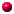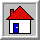﻿ Congjun Wu's homepage Congjun Wu's homepage

Quaternionic analyticity and high dimensional SU(2) Landau levels

##Quaternion

Quaternion (also known as Hamilton number), is an extension of complex number, and the first discovered non-commutive division algebra by Hamilton. It is spanned by three anti-communting imaginary units i, j, k, satisfying i^2=j^2=k^2=-1, and ij=-ji=k, jk=-kj=i, and ki=-ik=j. Quanternionic analyticity is a high dimensional generalization of the complex analyticity, described by the Cauchy-Riemann-Feuter condition, which is an Eucliean version of the Weyl equation.

##Quaternionic analytic Landau levels

We find a deep connection between quaternionic analyticity and high dimensional topological states [Ref. 1] . The Landau levels are generalzed to 3D and 4D possessing the full high dimensional rotation symmetry and flat dispersion by employing the SU(2) Aharanov-Casher potential. In analogy to the complex analyticity which had facilitated the study of 2D fractional quantum Hall states, we proved that the 3D and 4D lowest Landau level wavefunctions exhibit beautiful analytic properties, satisfying the Cauchy-Riemann-Fueter condition of the quaternionic analyticity. We expect that the quaternionic analyticity would provide a guiding principle for constructing high dimensional fractional topological states, which is a major challenging open question in the field, and the 3D LLs provide a possible platform for this research. In contrast, most 3D topological insulator models are based on lattice systems exhibiting complicated Bloch wavefunctions and dispersive energy spectra, both of which are obstacles for studying 3D fractional topological states.

The 3D lowest Landau level (LLL) wavefunctions are most intuitively explained in the coherent state representation: Pick up an arbitrary right-handed triad with three orthogonal axes e_1, e_2, and e_3. The LLL wavefunctions are analytic functions in the complex plane of e_1 + i e2 with spin polarized along the e_3 axis. The coupling between the orbital chirality and spin polarization forms a helicity structure, hence, the time-reversal symmetry is maintained. Its wavefunction analyticity exhibits in the rigid-body configuration space, or the SU(2) group space, and is best represented by quaternions. Each Landau level also contributes a branch of helical Dirac surface modes on the open boundary. We also constructed the 3D and 4D Landau levels based on the Landau-type gauge with flat bulk spectra and topologically non-trivial surface states [Ref 2.] . For the 4D case, the system exhibits quantized non-linear electromagnetic response as a spatially separated (3+1)D chiral anomaly.

##High dimensional Landau levels of Dirac fermions

We further constructed the 3D LLs for Dirac electrons, whose zeroth Landau level states are a flat band of the half-fermion Jackiw-Rebbi zero modes [Ref. 3] . This can be viewed as a squre-root problem of the above high dimensional Landau levels for non-relativistic fermions. It is at the interface between condensed matter and high energy physics, related to a new type of anomaly. Unlike parity anomaly and chiral anomaly studied in field theory in which Dirac fermions couple to gauge fields through the minimal coupling, here Dirac fermions couple to the background field in a non-minimal way.

References and talks
• 1. Yi Li, Congjun Wu, "High-Dimensional Topological Insulators with Quaternionic Analytic Landau Levels",
Phys. Rev. Lett. 110, 216802 (2013)
. See pdf file .

• 2. Yi Li, Shou-Cheng Zhang, Congjun Wu, "Topological insulators with SU(2) Landau levels",
Phys. Rev. Lett. 111, 186803 (2013)
. See pdf file

• 3. Yi Li, Kenneth Intriligator, Yue Yu, Congjun Wu, "Isotropic Landau levels of Dirac fermions in high dimensions",
Phys. Rev. B 85, 085132 (2012) , see pdf file.

• Talk: "Quaternionic analyticity and SU(2) Landau Levels in 3D"Back to home